Start Now with 100 Free Questions

# CogAT 6th Grade Practice Test

### 1.) Quantitative Battery: Number Puzzles

In this section, you will be given a mathematical equation. Choose the answer that should replace the ? or the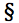symbol.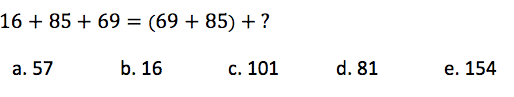### 2.) Non-Verbal Battery: Figure Classification

Parent say to your child: Tell your child to look at the first three figures. They go together in some way. Choose one more figure in the rest of the row that goes with the first three figures in the same way.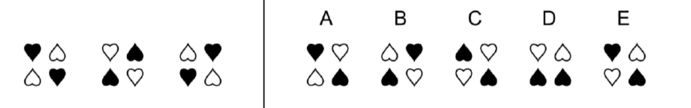### 3.) Verbal Battery: Verbal Analogy

The first two words in the sentences below go together in a certain way. Choose the answer that goes with the third word in the sentence the same way that the first and second words go together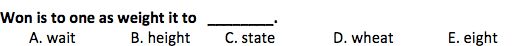### 4.) Quantitative Battery: Number Series

Look at the numbers in each row below. There is a rule that governs the order in which the numbers occur in the series. Figure out the rule and then choose the number that comes next from the answer choices below.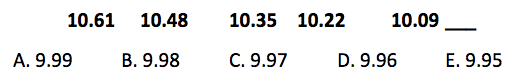### 5.) Non-Verbal Battery: Paper Folding

On the top row you will see how a square piece of paper is folded sometimes once, sometimes twice, sometimes three times. Holes are then punched after the paper has been folded. Choose the answer in the second row that shows how the folded piece of paper would look after it is unfolded.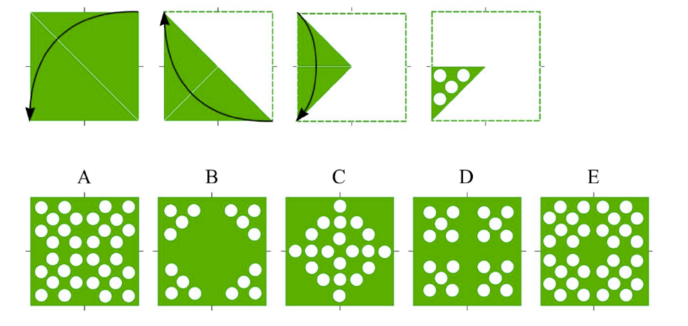1.)b. 16

2.) C- The exact same figures are always diagonally across from each other

3.) A

4.) D. 9.96 (Subtract 0.13)

5.) E

7 Responses

kasarlaruchir

Great to study for a GT test. Just wish there were more free practice tests….

swikina

Great!!

renxiaorui1234

great!

akul.ramesh.030

Thank you beta

Lindsey

Great!!

novsatishr48

i wish their were more practice tests

Akanksha

Looking for CogAT 6th Grade Practice Test